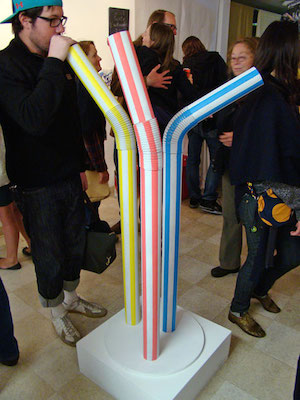# Longest strawI drink from a glass of water with a vertical straw. What's the longest straw I can use and still drink water if the ambient pressure is $1~\mbox{atm}$? Give your answer in meters.


Details and Assumptions:

• $1~\mbox{atm}=101,325~\mbox{Pa}$.
• The acceleration of gravity is $-9.8~\mbox{m/s}^2$.
• The density of water is $1~\mbox{g/cm}^3$.
×